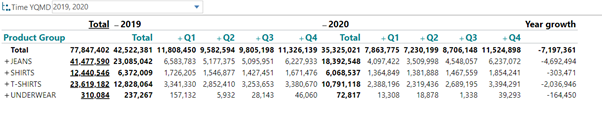# Addressing levels in hierarchies #1

When you work with hierarchies, you often need to address a certain level of the hierarchy and that can be a little difficult.

Let me start by showing you a few examples:

In this crosstab I would like to create a calculate the difference between the 2 year in the filter.As you can see – it’s a hierarchy of time that we are looking at on the horizontal axis.
That hierarchy is currently expanded to the quarter level – but I guess the users might expand it further (to month/day level).

However the hierarchy is expanded, I want to stick with the year level.

My time hierarchy has 4 levels: Year, Quarter, Month, Day

In terms of TARGIT syntax we can address:

• Level 0 (means the grand total of the time dimension – in this case the sum of 2019 and 2020)
• Level 1 (means year level – in this case either the sum of 2019 and the sum of 2020)
• Level 2 (means month level – in this case either the sum of Q1, Q2, Q3 and Q4)
• Level 3 (means day level) – in this case a particular day in a particular month)

This formula (Year growth)

Sum(d2(l1),0,m1)-sum(d1(l1),0,m1) means subtract 2019 from 2020 on year level.Because of the l1 reference this will remain on year level regardless how the hierarchy is expanded.

2 out of 2 found this helpful

1 comment
• Is there anything to be aware of when calculating with levels? I can get it to work on coded measures but not calculated measures.

The table contains sales data for the past 12 months, date hierarchy used is YMD(Month) thus the same as the example.

I want the total for this year only.

It gives me the correct numbers if I use specific dimensions:

c1 = (@".[January]":d-1, 0, m4) = correct
but this is very unconvenient as it requieres me to remember to change it next year.

It does not work with calculated measure m4:
m4 = returns m1 if store is older than 1 year else 0

c1 = d2(l1),0,m4) = 0 (not correct)

But if

c1 = d2(l1),0,m1) = returns turnover for 2022
which means it works, just not usable in the wanted context.

I also tried to use a different calculated measure to see what happens:

m3 = 1

c1: sum(d2(l1), 0, m3) = 1
but should be 7  so this does something, but does not calculate the total sum of 2022 for m3, looks like it takes the average rather than sum in this instance.

Any suggestions?